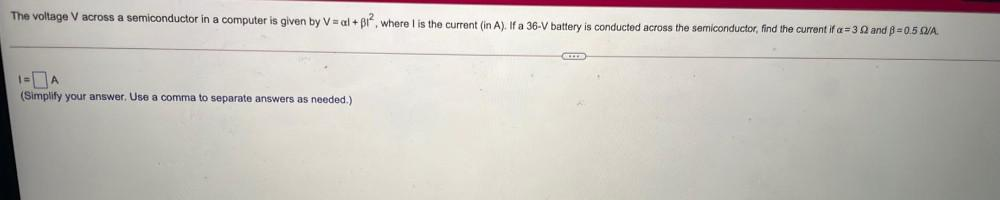Question:

# The voltage Vacross a semiconductor in a computer is given by V = al + B12, where is the current (in A). If a 36-V battery is coThe voltage Vacross a semiconductor in a computer is given by V = al + B12, where is the current (in A). If a 36-V battery is conducted across the semiconductor, find the current if a=32 and = 0.5 QUA. 1A (Simplify your answer. Use a comma to separate answers as needed.)